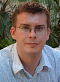Aggregating the Result of an MDX Calculation Using Scoped Assignments

Reposted from Chris Webb's blog with the author's permission.

I don't usually like to blog about topics that I think other people have blogged about already, but despite the fact that Mosha blogged about this several years ago (in fact more than eight years ago, blimey) this particular problem comes up so often with my customers and on the MSDN Forum that I thought I should write something about it myself. So apologies if you know this already.

Here's the problem description. If you define a calculated measure in MDX, that calculation will take place after the real measure values have all aggregated. For example, consider a super-simple cube with a Year dimension, two real measures called A and B and a calculated measure called [A * B] that returned the value of A multiplied by B. In a PivotTable you'd see the following result:Note how the Grand Total for the [A * B] calculated measure is 12*16=192, and not 12+12+12+12=48. This is expected behaviour for calculated measures, and indeed the way you want your calculations to behave most of the time.

However, there are some scenarios where you want to do the calculation first and then aggregate up the result of that calculation; in our previous example that means you'd get 48 for the Grand Total instead. Currency conversion and weighted averages are common examples of calculations where this needs to happen. How can you handle this in MDX?

Let's look at a slightly more complex example than the one above. In the following cube, based on Adventure Works data, I created a Date dimension that looks like this:.and a Product dimension that looks like this:I also created two measures on a fact table called A and B:Now, let's say that once again you want to calculate the value of A*B at the Date and Product granularity, and aggregate the result up. Probably the easiest way of handling this would be to do the calculation in the fact table, or in the DSV, and then bringing the result in as a new real measure. However this may not be possible with some types of calculation, or if the granularity that you want to do the calculation is not the same as the granularity of the fact table.

One way of approaching this in MDX would be to create a calculated measure like this:

CREATE MEMBER CURRENTCUBE.MEASURES.[CALC] AS
SUM(
DESCENDANTS([Date].[Calendar].CURRENTMEMBER, [Date].[Calendar].[Date])
*
DESCENDANTS([Product].[Category - Product].CURRENTMEMBER, [Product].[Category - Product].[Product])
, [Measures].[A] * [Measures].[B]);The big problem with this approach (apart from the fact that it may break when you do a multi-select in certain client tools - but you could work around that) is that it is usually very, very slow indeed. Depending on the calculation, it may be unusably slow. So you need a different approach.

This is where scoped assignments come in. If you make a scoped assignment to a real measure, as opposed to a calculated measure, then the value of the assignment will aggregate up outside of the original scope. So, in this case, since you want the calculation to take place at the Date and Product granularity, if you scope on a real measure at that granularity the result of the calculation will aggregate up automatically.

The first step here is to create a new real (ie not calculated) measure for the calculation. This can be done in the DSV by creating a named calculation on your fact table which returns the value NULL:You then need to create a new real measure on your measure group from this new named calculation:In this example, I've left the AggregateFunction property of the measure to be the default of Sum, but you could use a different setting if you wanted a different type of aggregation. The next step is to process the cube, and once you've done that you'll see a new measure that only returns the value 0:Next, you need to create the scoped assignment on the Calculations tab of the Cube Editor. If you remember in my post last week about scoped assignments, I recommended avoiding writing scopes using user hierarchies; using only attribute hierarchies the scope statement becomes:

SCOPE([Measures].[A Multiplied By B]);
SCOPE([Date].[Date].[Date].MEMBERS);
SCOPE([Product].[Product].[Product].MEMBERS);
THIS = [Measures].[A] * [Measures].[B];
END SCOPE;
END SCOPE;
END SCOPE;One very important thing to notice: the sets I've used for scoping on the Dates and Products do not include the All Member: for example, [Date].[Date].[Date].MEMBERS. If you use a set that includes the All Member, such as [Date].[Date].MEMBERS, the calculation will not aggregate up correctly.

Here's the result:This is going to be much more efficient than the pure MDX calculated measure approach, though just how well the calculation performs will depend on the complexity of the calculation and the size of the area that you are scoping on.Chris has been working with Microsoft BI tools since he started using beta 3 of OLAP Services back in the late 90s. Since then he has worked with Analysis Services in a number of roles (including three years spent with Microsoft Consulting Services) and he is now an independent consultant specialising in complex MDX, Analysis Services cube design and Analysis Services query performance problems. His company website can be found at http://www.crossjoin.co.uk and his blog can be found at http://cwebbbi.wordpress.com/ .

Tags: mdx, performance

2007-2015 VidasSoft Systems Inc.